## Build a Markdown Previewer with Vue.js

Learn how to build a markdown previewer with Vue.js in this tutorial for beginners.

🖥️ Code: sorry, I lost my code files and forgot to commit them to github… THIS IS WHY YOU COMMIT OFTEN!

🔗Check out Cody Seibert’s main channel: https://www.youtube.com/channel/UCsrVDPJBYeXItETFHG0qzyw

Read hundreds of articles on programming: https://medium.freecodecamp.org

source

### This Post Has 12 Comments

1.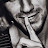First watching

2.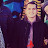Hey Cody, the Github repo is empty….

3.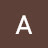Thank u for this awesome video

4.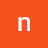More project Vuejs, pleaseeeeeeeeee Cody 😭😭😭😭

5.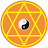Nice Vue.js coding tutorial, thank you!

6.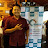but how to handle a code in markdownfile?

7.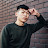Hoooooo

8.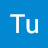9.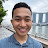Thank you this is what I've been trying to do for so long!

10.For those who wants the code…

const rules = [

[/#{6}s?([^n]+)n/g, '<h6>\$1</h6>'],

[/#{5}s?([^n]+)n/g, '<h5>\$1</h5>'],

[/#{4}s?([^n]+)n/g, '<h4>\$1</h4>'],

[/#{3}s?([^n]+)n/g, '<h3>\$1</h3>'],

[/#{2}s?([^n]+)n/g, '<h2>\$1</h2>'],

[/#{1}s?([^n]+)n/g, '<h1>\$1</h1>'],

[/([^n]+)ns+=+/g, '<h1>\$1</h1><hr/>'],

[/([^n]+)ns+-+/g, '<h2>\$1</h2><hr/>'],

[/**([^*n]+)**/g, '<b>\$1</b>'],

[/*([^*n]+)*/g, '<i>\$1</i>'],

[/__([^_]+)__/g, '<b>\$1</b>'],

[/_([^_]+)_/g, '<i>\$1</i>'],

[/((nd..+)+)/g, '<ol>\$1</ol>'],

[/((n*.+)+)/g, '<ul>\$1</ul>'],

[/nd.([^n]+)/g, '<li>\$1</li>'],

[/n*([^n]+)/g, '<li>\$1</li>'],

[/![([^]]+)](([^)]+)s"([^")]+)")/g, '<img src>…'],

[/{([^]]+)](([^)]+))/g, '<a href="\$2">\$1</a>'],

[/([^n]+n)/g, '<p>\$1</p>']

]

export default {

bind(el) {

let html = el.textContent;

rules.forEach(([rule, template]) => {

html = html.replace(rule, template);

})

el.innerHTML = html;

}

}

11.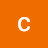why don't you make a video with the materials we have learned so far in my FCC?

12.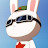This is an amazing video. Short but powerful!